Finite Mathematics : Mathematics of Finance

Example Questions

Example Question #1 : Mathematics Of Finance

What is the total interest made after 4 years on a simple interest loan that accumulates 13% each year and has original amount was $1350. Possible Answers: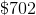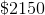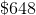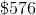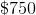Correct answer:Explanation: To solve this problem, first recall the formula to calculate simple interest.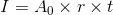where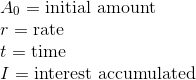From the question it's known that,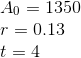Thus the equation becomes,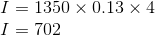Therefore$702 in interested accumulated after four years.

Example Question #2 : Mathematics Of Finance

Money is deposited in a bank account at 7% interest per year, compounded bimonthly. If no money is deposited into or withdrawn from the account, then how long will it take for the money to double?

9 years 8 months

10 years 2 months

9 years 10 months

9 years 6 months

10 years 0 months

10 years 0 months

Explanation:

If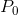is deposited in a bank account with interest rate( converted to a decimal) compoundedtimes a year foryears, then the final balance of the account is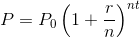Dividing both sides of the formula by, this is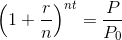The money doubles, so substitute 2 for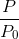. Also, set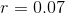and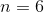(bimonthly), and calculateas follows: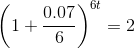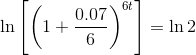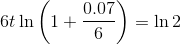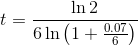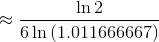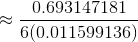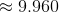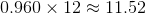, so this is 9 years and 11.52 months; rounding up to the next bimonth, this is 10 years even.